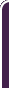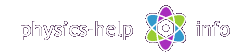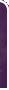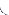homephysics online guidephysics help my info terms of use contactsWhat is Physics? Mechanics Electricity and Magnetism Electric Field Gauss' Law about below subjects Electric Potential Capacity Direct Current Magnetic Field Magnetic Field Laws Magnetic Interactions Electromagnetic Induction Maxwell's Equations Oscillations and Waves Simple Harmonic Motion Damped Harmonic Motion Driven Harmonic Motion Electric Oscillation Alternating Current Wave Motion Elastic Waves Electromagnetic Waves Optics Light Waves Geometrical Optics Interference Polarization Diffraction Fraunhofer Diffraction Dispersion, Absorption, Diffusion Doppler Effect Thermodynamics Ideal Gas Molecular Statistics Transport Phenomena First Law of Thermodynamics Second and Third Laws of Thermodynamics Imperfect Gas Liquids Solids Quantum Physics Thermal Radiation Quantum Properties of Light Wave Properties of Particles Planetary Model of Atom X-Rays Particle in Potential Well Pauli Exclusion Principle Nuclear Physics Solid State Physics Appendices# Physics Constants used in the Guide

Mechanics:

Gravitational constant,Volumetric mean radius of Earth,Mass of Earth,Mean free-fall acceleration at the Earth's surface,Electricity and Magnetism:

Elementary charge,Permittivity of vacuum,Permeability of vacuum,Coulomb constant,Speed of light in vacuumStatistical Physics and Thermodynamics:

Avogadro constant,Boltzmann constant,Gas constant,Standard atmospheric conditions:Quantum Physics:

Stefan-Boltzmann constant,Planck constant,,Electron mass,Proton mass,Neutron mass,Rydberg constant,Bohr radius,Hydrogen ground state,Compton wavelength,Bohr magneton,Atomic mass unit,Electron-volt,home  |  physics online guide  |  physics help  |  my info  |  terms of use  |  contacts Dr. Nikitin © 2010